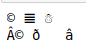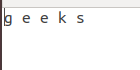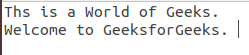# Working with Binary Data in Python

Alright, lets get this out of the way! The basics are pretty standard:

1. There are 8 bits in a byte
2. Bits either consist of a 0 or a 1
3. A byte can be interpreted in different ways, like binary octal or hexadecimal

Note: These are not character encodings, those come later. This is just a way to look at a set of 1’s and 0’s and see it in three different ways(or number systems).

Examples:

```Input : 10011011

Output :
1001 1011 ---- 9B (in hex)
1001 1011 ---- 155 (in decimal)
1001 1011 ---- 233 (in octal)
```

This clearly shows a string of bits can be interpreted differently in different ways. We often use the hex representation of a byte instead of the binary one because it is shorter to write, this is just a representation and not an interpretation.

## Encoding

Now that we know what a byte is and what it looks like, let us see how it is interpreted, mainly in strings. Character Encodings are a way to assign values to bytes or sets of bytes that represent a certain character in that scheme. Some encodings are ASCII(probably the oldest), Latin, and UTF-8(most widely used as of today. In a sense encodings are a way for computers to represent, send and interpret human readable characters. This means that a sentence in one encoding might become completely incomprehensible in another encoding.

## Python and Bytes

From a developer’s point of view, the largest change in Python 3 is the handling of strings. In Python 2, the str type was used for two different kinds of values – text and bytes, whereas in Python 3, these are separate and incompatible types. This means that before Python3 we could treat a set of bytes as a string and work from there, this is not the case now, now we have a separate data type, called bytes. This data type can be briefly explained as a string of bytes, which essentially means, once the bytes data type is initialized it is immutable.

Example:

## Python3

 `bytestr ``=` `bytes(b``'abc'``)  ` ` `  `# initializing a string with b ` `# makes it a binary string ` `print``(bytestr) ` `print``(bytestr[``0``]) ` ` `  `bytestr[``0``] ``=` `97`

Output:

```b'abc'
97
Traceback (most recent call last):
File "bytesExample.py", line 4, in
bytestr = 97
TypeError: 'bytes' object does not support item assignment
```

A bytestring is what it says it is simply a string of bytes, for example ‘© ? ?’ in ‘utf-8’ is

```b'\xC2\xA9\x20\xF0\x9D\x8C\x86\x20\xE2\x98\x83'
```

This presents another problem, we need to know the encoding of a binary string, because the same string in another encoding(latin-1) looks different.

```Â© ð â
```

Example:

## Python3

 `print``(b``'\xC2\xA9\x20\xF0\x9D\x8C\x86\x20\xE2\x98\x83'``.decode(``'utf-8'``)) ` `print``(b``'\xC2\xA9\x20\xF0\x9D\x8C\x86\x20\xE2\x98\x83'``.decode(``'latin-1'``))`

Output:As seen above it is possible to encode or decode strings and binary strings using the encode() or decode() function. We need the encoding because in some encodings it is not possible to to decode the strings. This problem compounds when not using non Latin characters like Hebrew, Japanese and Chinese. Because in those languages more than one byte is assigned to each letter. But what do we use when we need to modify a set of bytes, we use a bytearray

Example:

## Python3

 `bytesArr ``=` `bytearray(b``'\x00\x0F'``) ` ` `  `# Bytearray allows modification ` `bytesArr[``0``] ``=` `255` `bytesArr.append(``255``) ` `print``(bytesArr)`

Output:

`bytearray(b'\xff\x0f\xff')`

## Bitwise Operations

In Python, bitwise operators are used to perform bitwise calculations on integers. The integers are first converted into binary and then operations are performed on bit by bit, hence the name bitwise operators. The standard bitwise operations are demonstrated below.

Example:

## Python3

 `# Code to demonstrate bitwise operations ` `# Some bytes to play with ` `byte1 ``=` `int``(``'11110000'``, ``2``)  ``# 240 ` `byte2 ``=` `int``(``'00001111'``, ``2``)  ``# 15 ` `byte3 ``=` `int``(``'01010101'``, ``2``)  ``# 85 ` ` `  `# Ones Complement (Flip the bits) ` `print``(~byte1) ` ` `  `# AND ` `print``(byte1 & byte2) ` ` `  `# OR ` `print``(byte1 | byte2) ` ` `  `# XOR ` `print``(byte1 ^ byte3) ` ` `  `# Shifting right will lose the  ` `# right-most bit ` `print``(byte2 >> ``3``) ` ` `  `# Shifting left will add a 0 bit  ` `# on the right side ` `print``(byte2 << ``1``) ` ` `  `# See if a single bit is set ` `bit_mask ``=` `int``(``'00000001'``, ``2``)  ``# Bit 1 ` ` `  `# Is bit set in byte1? ` `print``(bit_mask & byte1) ` ` `  `# Is bit set in byte2? ` `print``(bit_mask & byte2)`

Output:

```-241
0
255
165
1
30
0
1```

## Some Other Applications

Binary data provides several applications like we can check if the two files are similar or not using the binary data, we can also check for a whether a file is jpeg or not (or any other image format). Let’s see the below examples for better understanding.

Example 1: Checking if the two files are same or not. Here two text files are used with the data as follows –

File 1:File 2:## Python3

 `with ``open``(``'GFG.txt'``, ``'rb'``) as file1, ``open``(``'log.txt'``, ``'rb'``) as file2: ` `    ``data1 ``=` `file1.read() ` `    ``data2 ``=` `file2.read() ` ` `  `if` `data1 !``=` `data2: ` `    ``print``(``"Files do not match."``) ` `else``: ` `    ``print``(``"Files match."``)`

Output:

`Files do not match.`

Example 2: Checking if the given image is jpeg or not.

Image used:## Python3

 `import` `binascii ` ` `  `jpeg_signatures ``=` `[ ` `    ``binascii.unhexlify(b``'FFD8FFD8'``), ` `    ``binascii.unhexlify(b``'FFD8FFE0'``), ` `    ``binascii.unhexlify(b``'FFD8FFE1'``) ` `] ` ` `  `with ``open``(``'food.jpeg'``, ``'rb'``) as ``file``: ` `    ``first_four_bytes ``=` `file``.read(``4``) ` ` `  `    ``if` `first_four_bytes ``in` `jpeg_signatures: ` `        ``print``(``"JPEG detected."``) ` `    ``else``: ` `        ``print``(``"File does not look like a JPEG."``)`

Output:

`JPEG detected.`

My Personal Notes arrow_drop_upCheck out this Author's contributed articles.

If you like GeeksforGeeks and would like to contribute, you can also write an article using contribute.geeksforgeeks.org or mail your article to contribute@geeksforgeeks.org. See your article appearing on the GeeksforGeeks main page and help other Geeks.

Please Improve this article if you find anything incorrect by clicking on the "Improve Article" button below.

Article Tags :

Be the First to upvote.

Please write to us at contribute@geeksforgeeks.org to report any issue with the above content.